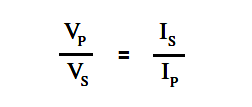# Transformer Current Ratio

The current in the windings of a transformer is inversely proportional to the voltage in the windings.

This relationship is expressed in below Equation.where

IP = primary coil current

IS = secondary coil current

Since the voltage ratio is equal to the turns ratio, we can express the current ratio in terms of the turns ratio, as in below Equation.Example 1:

When operated at 120 V in the primary of an iron core transformer, the current in the primary is 4 amps. Find the current in the secondary if the voltage is stepped up to 500 V.

Solution:Next, we solve for Is

Is = (Vp/Vs) Ip

Is = (120/500) 4

Is = 0.96 amps

Example 2:

A transformer with 480 turns on the primary and 60 turns on the secondary draws Solution: 0.6 amps from a 120 V line. Find Is.

Solution :Next, we solve for Is

Is = (Np/Ns) Ip

Is = (480/60) 0.6

Is = 4.8 amps

We should note from the previous examples that a transformer that “steps-up” voltage, “steps-down” the current proportionally.